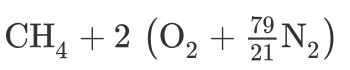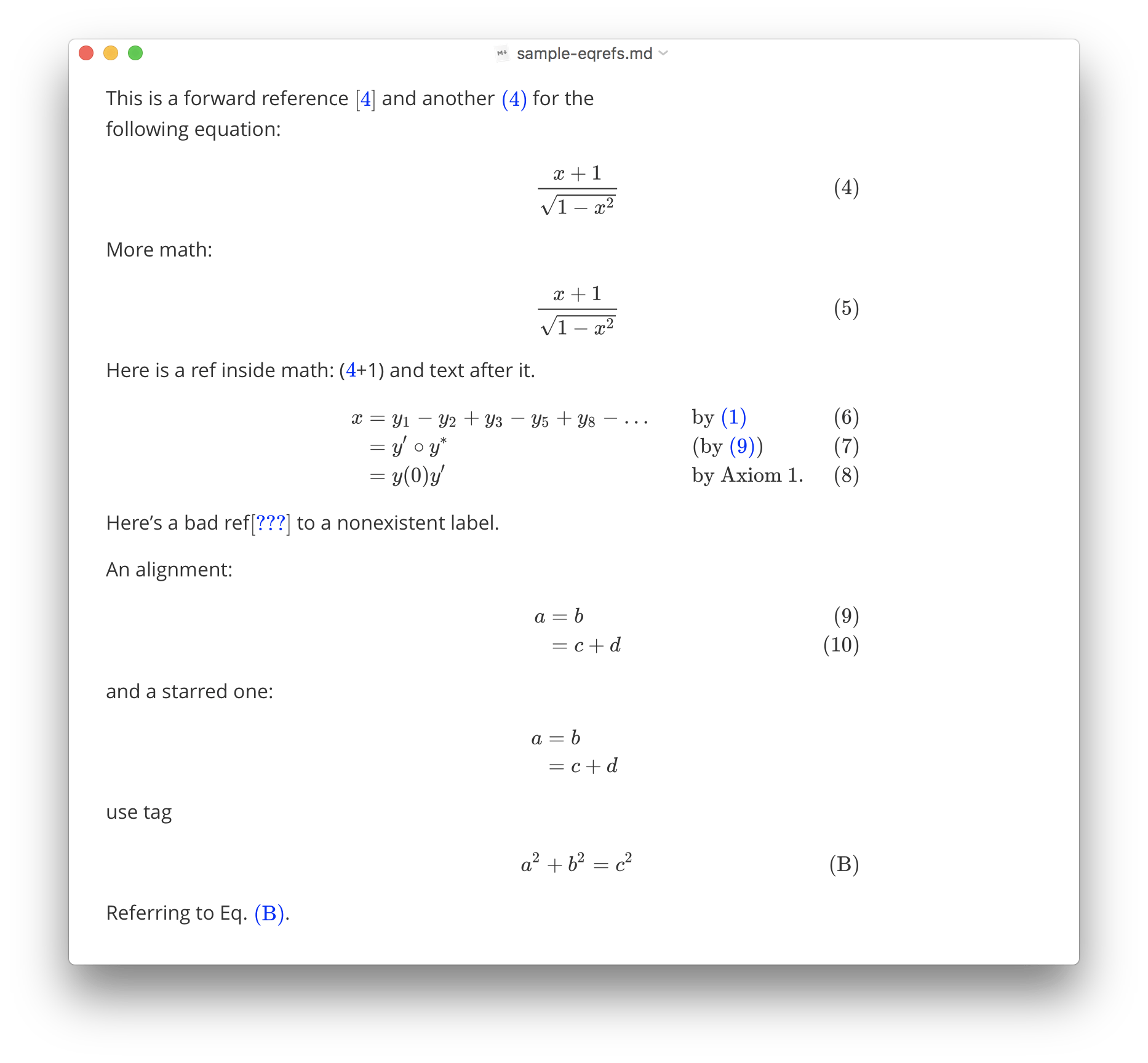Typora supports rendering normal mathematics using Tex/LaTeX syntax. The rendering process is processed by MathJax.

## Math Block (Display Math)

Math blocks are LaTeX expressions wrapped by $$ mark and line break, for example: $$
\begin{align*}
y = y(x,t) &= A e^{i\theta} \\
&= A (\cos \theta + i \sin \theta) \\
&= A (\cos(kx - \omega t) + i \sin(kx - \omega t)) \\
&= A\cos(kx - \omega t) + i A\sin(kx - \omega t)  \\
&= A\cos \Big(\frac{2\pi}{\lambda}x - \frac{2\pi v}{\lambda} t \Big) + i A\sin \Big(\frac{2\pi}{\lambda}x - \frac{2\pi v}{\lambda} t \Big)  \\
&= A\cos \frac{2\pi}{\lambda} (x - v t) + i A\sin \frac{2\pi}{\lambda} (x - v t)
\end{align*}
 will be rendered as \begin{align*} y = y(x,t) &= A e^{i\theta} \\ &= A (\cos \theta + i \sin \theta) \\ &= A (\cos(kx - \omega t) + i \sin(kx - \omega t)) \\ &= A\cos(kx - \omega t) + i A\sin(kx - \omega t) \\ &= A\cos \Big(\frac{2\pi}{\lambda}x - \frac{2\pi v}{\lambda} t \Big) + i A\sin \Big(\frac{2\pi}{\lambda}x - \frac{2\pi v}{\lambda} t \Big) \\ &= A\cos \frac{2\pi}{\lambda} (x - v t) + i A\sin \frac{2\pi}{\lambda} (x - v t) \end{align*} In typora, you could just press  and Return key to input a math block, in input mode, use Up/Down arrow key or Command/Ctrl + Return key to finish editing, or just click the ✓ button, or somewhere else.

## Inline Math

First of all, please enable inline math feature from preferences panels -> Markdown section. Preferences panel can be opened From menu bar: File -> Preferences..., or use shortcut key — command/ctrl + ,. This setting will be applied after Typora restarts.Inline math use syntax like this $<Math Expressions>$, e.g: $f = \frac{2 \pi}{T}$.

## TeX Commands available in Typora

You could find the all supported TeX commands in http://docs.mathjax.org/en/latest/input/tex/macros/index.html.

You could add new commands using \def or\newcommand. For example:

$$\def\bold#1 \bold{this\ is\ now\ bold}$$


## Chemistry Expressions

Typora has the built-in mhchem extension, which can be used to render Chemistry Expressions, you could use like this:

$\ce{CH4 + 2$\left( \ce{O2 + 79/21 N2} \right)$}$


Which will be rendered asFor more details, please refer https://mhchem.github.io/MathJax-mhchem/.

## Cross Reference

Typora supports TeX-style reference syntax, for example:

Here is a labeled equation:

$$x+1\over\sqrt{1-x^2}\label{ref1}\tag{1}$$

This is a reference : $\ref{ref1}$


## Auto Numbering

Typora supports auto-numbering math blocks. To turn on this feature, please open preferences panel, and enable “Auto Numbering Math Equations” under the “Markdown” section.## Troubleshooting

### Force Refresh

When Math rendering goes wrong, like output math too wild/narrow, or equation numbering becomes incorrect, you can trigger force refresh for all math from the EditMath Tools menu.

### Line Breaking

Starting from v0.11.0, Typora upgraded to MathJax v3, which does not support line break using \\ or \newline in MathJax v2, the behavior is same with LaTeX, unless you put them in a \displaylines{} environment, for example:

\displaylines{x+y\\y+z}


So you could use \displaylines as a workaround.

From v0.11.1, Typora provides a new option “Apply Line Break at \\” under Preferences PanelMarkdownMath. By enabling it, typora will auto wrap your math content with \displaylines if your content contains \\ or \newline to achieve the same behavior as previous versions. But it may bring some unexpected issues, so if you met any math issues after enabled it, please try disable the option, and report to us, if it is the cause.

Use \displaylines instead of enabling “Apply Line Break at \\” is recommended for adding line breaks in math.